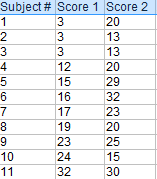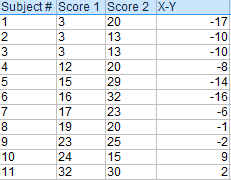# T Test (Student’s T-Test): Definition and Examples

T Test: Contents:

## What is a T test?

Watch the video for an overview of the T-test:

The t test tells you how significant the differences between group means are. It lets you know if those differences in means could have happened by chance. The t test is usually used when data sets follow a normal distribution but you don’t know the population variance.

For example, you might flip a coin 1,000 times and find the number of heads follows a normal distribution for all trials. So you can calculate the sample variance from this data, but the population variance is unknown. Or, a drug company may want to test a new cancer drug to find out if it improves life expectancy. In an experiment, there’s always a control group (a group who are given a placebo, or “sugar pill”). So while the control group may show an average life expectancy of +5 years, the group taking the new drug might have a life expectancy of +6 years. It would seem that the drug might work. But it could be due to a fluke. To test this, researchers would use a Student’s t-test to find out if the results are repeatable for an entire population.

In addition, a t test uses a t-statistic and compares this to t-distribution values to determine if the results are statistically significant.

However, note that you can only uses a t test to compare two means. If you want to compare three or more means, use an ANOVA instead.

## The T Score.

The t score is a ratio between the difference between two groups and the difference within the groups.

• Larger t scores = more difference between groups.
• Smaller t score = more similarity between groups.

A t score of 3 tells you that the groups are three times as different from each other as they are within each other. So when you run a t test, bigger t-values equal a greater probability that the results are repeatable.

### T-Values and P-values

How big is “big enough”? Every t-value has a p-value to go with it. A p-value from a t test is the probability that the results from your sample data occurred by chance. P-values are from 0% to 100% and are usually written as a decimal (for example, a p value of 5% is 0.05). Low p-values indicate your data did not occur by chance. For example, a p-value of .01 means there is only a 1% probability that the results from an experiment happened by chance.

## Calculating the Statistic / Test Types

There are three main types of t-test:

You can find the steps for an independent samples t test here. But you probably don’t want to calculate the test by hand (the math can get very messy. Use the following tools to calculate the t test:

## What is a Paired T Test (Paired Samples T Test / Dependent Samples T Test)?

A paired t test (also called a correlated pairs t-test, a paired samples t test or dependent samples t test) is where you run a t test on dependent samples. Dependent samples are essentially connected — they are tests on the same person or thing. For example:

• Knee MRI costs at two different hospitals,
• Two tests on the same person before and after training,
• Two blood pressure measurements on the same person using different equipment.

## When to Choose a Paired T Test / Paired Samples T Test / Dependent Samples T Test

Choose the paired t-test if you have two measurements on the same item, person or thing. But you should also choose this test if you have two items that are being measured with a unique condition. For example, you might be measuring car safety performance in vehicle research and testing and subject the cars to a series of crash tests. Although the manufacturers are different, you might be subjecting them to the same conditions.

With a “regular” two sample t test, you’re comparing the means for two different samples. For example, you might test two different groups of customer service associates on a business-related test or testing students from two universities on their English skills. But if you take a random sample each group separately and they have different conditions, your samples are independent and you should run an independent samples t test (also called between-samples and unpaired-samples).

The null hypothesis for the independent samples t-test is μ1 = μ2. So it assumes the means are equal. With the paired t test, the null hypothesis is that the pairwise difference between the two tests is equal (H0: µd = 0).

## Paired Samples T Test By hand

Example question: Calculate a paired t test by hand for the following data:Step 1: Subtract each Y score from each X score.Step 2: Add up all of the values from Step 1 then set this number aside for a moment.1. The “ΣD” is the sum of X-Y from Step 2.
2. ΣD2: Sum of the squared differences (from Step 4).
3. (ΣD)2: Sum of the differences (from Step 2), squared.

If you’re unfamiliar with the Σ notation used in the t test, it basically means to “add everything up”. You may find this article useful: summation notation.

Step 6: Subtract 1 from the sample size to get the degrees of freedom. We have 11 items. So 11 – 1 = 10.

Step 7: Find the p-value in the t-table, using the degrees of freedom in Step 6. But if you don’t have a specified alpha level, use 0.05 (5%).

So for this example t test problem, with df = 10, the t-value is 2.228.

Step 8: In conclusion, compare your t-table value from Step 7 (2.228) to your calculated t-value (-2.74). The calculated t-value is greater than the table value at an alpha level of .05. In addition, note that the p-value is less than the alpha level: p <.05. So we can reject the null hypothesis that there is no difference between means.

However, note that you can ignore the minus sign when comparing the two t-values as ± indicates the direction; the p-value remains the same for both directions.

In addition, check out our YouTube channel for more stats help and tips!

## References

Goulden, C. H. Methods of Statistical Analysis, 2nd ed. New York: Wiley, pp. 50-55, 1956.

CITE THIS AS:
Stephanie Glen. "T Test (Student’s T-Test): Definition and Examples" From StatisticsHowTo.com: Elementary Statistics for the rest of us! https://www.statisticshowto.com/probability-and-statistics/t-test/